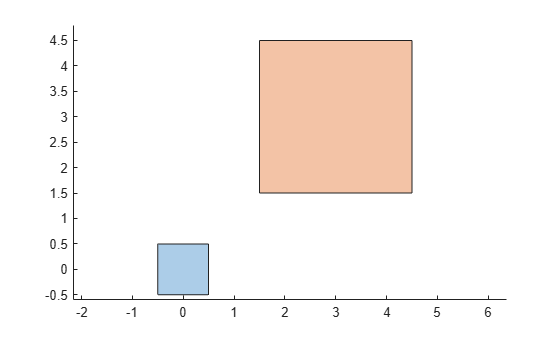turningdist

Compute turning distance between polyshape objects

Description

td = turningdist(poly1,poly2) returns an array of turning distances between the corresponding element pairs of two polyshape arrays with compatible sizes. The turning distance between two polyshape objects is a measure of how closely their shapes match, regardless of rotation or scaling. A turning distance close to 0 indicates a near match. The larger the value, the more the two shapes differ.

TD(i,j) is the turning distance between the ith polyshape in poly1 and the jth polyshape in poly2.

example

td = turningdist(polyvec) returns a matrix of turning distances between element pairs of a vector of polyshape objects.

Examples

collapse all

Create and plot two squares of different sizes and locations, represented as polyshape objects poly1 and poly2.

poly1 = nsidedpoly(4,'SideLength',1);
poly2 = nsidedpoly(4,'SideLength',3,'Center',[3 3]);
plot(poly1)
hold on
plot(poly2)
axis equal
hold offBecause the two squares have the same shape despite their scaling, their turning distance is 0.

td = turningdist(poly1,poly2)
td = 0

Create and plot a third polyshape, and compare its turning distance to poly1. Since their shapes have more differences than poly1 and poly2, the turning distance is larger.

poly3 = nsidedpoly(20,'Center',[3 3]);
plot(poly1)
hold on
plot(poly3)
axis equal
hold offtd = turningdist(poly1,poly3)
td = 0.4443

Input Arguments

collapse all

First input polyshape, specified as a scalar, vector, matrix, or multidimensional array.

Second input polyshape, specified as a scalar, vector, matrix, or multidimensional array.

polyshape vector.

Output Arguments

collapse all

Turning distance, returned as a scalar, vector, matrix, or multidimensional array whose elements are greater than or equal to 0.

• If you input two polyshape arguments, then they must have compatible sizes. For example, if two input polyshape vectors have different lengths M and N, then they must have different orientations (one must be a row vector and one must be a column vector). td is then M-by-N or N-by-M depending on the orientation of each input vector. For more information on compatible array sizes, see Compatible Array Sizes for Basic Operations.

• If you input a single polyshape vector with length N, then td is N-by-N.

Data Types: double

 Arkin, E.M., Chew, L.P., Huttenlocher, D.P., Kedem, K., and Mitchell, J.S.B. "An efficiently computable metric for comparing polygonal shapes." IEEE Transactions on Pattern Analysis and Machine Intelligence. Vol. 13, Number 3, 1991, pp. 209-16. doi:10.1109/34.75509.